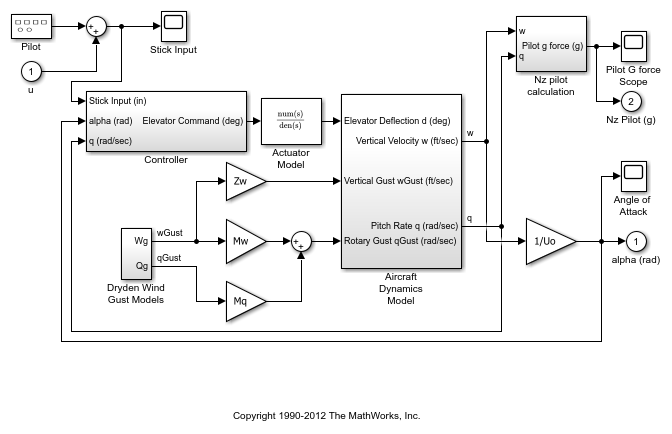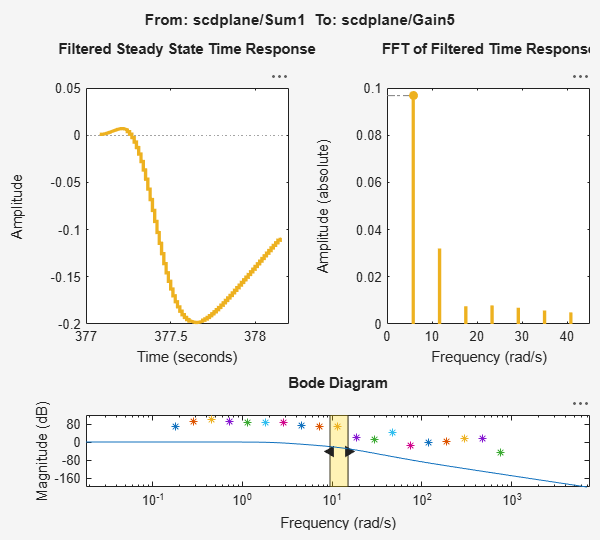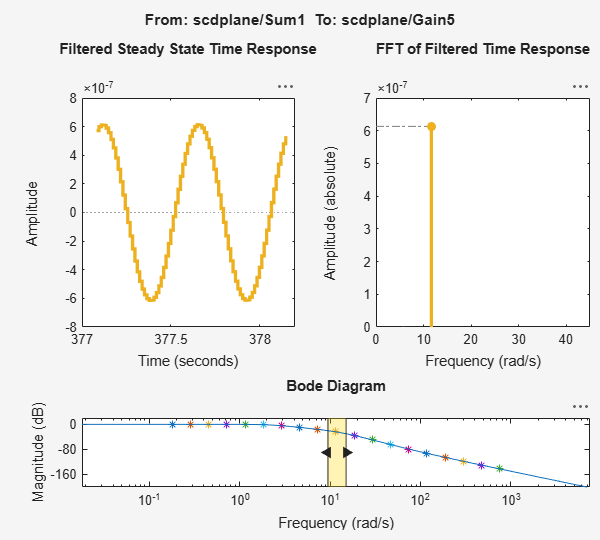# Disable Noise Sources During Frequency Response Estimation

This example shows how to disable noise sources in your Simulink® model during frequency response estimation. Such noise sources can interfere with the signal at the linearization output points and produce inaccurate estimation results.

Open the model.

```mdl = 'scdplane'; open_system(mdl) ```Specify linearization input and output points.

```io(1) = linio('scdplane/Sum1',1); io(2) = linio('scdplane/Gain5',1,'output'); ```

Linearize the model and create a sinestream estimation input signal based on the dynamics of the resulting linear system.

```sys = linearize(mdl,io); in = frest.Sinestream(sys); ```

Estimate frequency response.

```[sysest,simout] = frestimate(mdl,io,in); ```

Compare the estimated frequency response to the exact linearization result.

```frest.simView(simout,in,sysest,sys) ```In the Bode Diagram, the estimated frequency response does not match the response of the exact linearization. This result is due to the effects of the Pilot and Wind Gust Disturbance blocks in the model. To view the effects of the noise on the time response at a given frequency, right-click the time response plot and make sure Show filtered steady state output only is selected.

Locate the source blocks in the model.

```srcblks = frest.findSources(mdl,io); ```

Repeat the frequency response estimation with the source blocks disabled.

```opts = frestimateOptions('BlocksToHoldConstant',srcblks); [sysest,simout] = frestimate(mdl,io,in,opts); frest.simView(simout,in,sysest,sys) ```The resulting frequency response matches the exact linearization results.